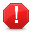# ExamplesThis documentation is for an older version of ZK. For the latest one, please click here.

### Single-Column Example : Using only setModel

In the following example, an array of data("data") is prepared , it is passed as a parameter for the generation of a ListModel("strset"). Last, assign this ListModel into a Grid by setting the model of the Grid.

```<window title="Live grid">
<zscript>
<![CDATA[
String[] data = new String;
for(int j=0; j < data.length; ++j) {
data[j] = "option "+j;
}
ListModel strset = new SimpleListModel(data);
]]>
</zscript>
<grid model="\${strset}">
<columns>
<column label="options" />
</columns>
</grid>
</window>
```

### Two-Columns Example : User defined renderer

Since the default rowrender only satisfies the scenario of single-column, a customized rowrenderer must be implemented to commit the scenario of multi-column. In the following example, a two-columns live data for grid is demonstrated.

#### 1.Prepare the Data Model

The first step is to prepare required data. A two-way array is adopted in this example and it is passed as a parameter for the generation of a ListModel which is implemented by SimpleListModel. In addition, ListModel now supports various data types,including Array, List, Map, and Set in the form of ListModelArray,ListModelList, ListModelMap,and ListModelSet.

```//prepare the data model
String[][] model = new String;
for(int j=0; j < model.length; ++j) {
model[j] = "key"+j;
model[j] = "value"+j;
}
ListModel strset = new SimpleListModel(model);
```

#### 2.Define the RowRenderer Class

Secondly, to define a class which implements the interface RowRenderer,its method render(Row row, java.lang.Object data) is required to be implemented.

```   Method render(Row row, java.lang.Object data)
row - The row to render the result.
data - Returned from ListModel.getElementAt()
```

In addition to pass required parameters into the method render(), the form of view (UI Component) for displaying data in the Grid also has to be defined, and any component which is supported by Grid could be adopted. In this example, Label is adopted, and the last step is to render Label into the specified row in the Grid by calling Label.setParent(Row row).

MyRowRenderer.java

```import org.zkoss.zul.Label;
import org.zkoss.zul.Row;
import org.zkoss.zul.RowRenderer;

//define the RowRenderer class
public class MyRowRenderer implements RowRenderer{

public void render(Row row, java.lang.Object data) {
String[] _data = (String[])data;
new Label(_data).setParent(row);
new Label(_data).setParent(row);
}
}
```

#### 3.Specify model and rowRenderer Property

The properties of model and rowRenderer could be specified directly in the Grid. In this example,methods, Grid.setModel() and Grid.setRowRenderer() are adopted to specify required objects for realizing the idea of "live data".

```<window title="Two-Column Live Data Demo" >
<grid id="mygrid">
<columns>
<column label="key" />
<column label="value" />
</columns>
</grid>
<zscript><![CDATA[
String[][] model = new String;

for(int j=0; j < model.length; ++j) {
model[j] = "key"+j;
model[j] = "value"+j;
}
ListModel strset = new SimpleListModel(model);
mygrid.setModel(strset);
mygrid.setRowRenderer(new MyRowRenderer());
]]>
</zscript>
</window>
```

Last Update : 2010/07/15